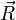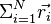# Ideal chain modelThis article is a 'stub' page, it has no, or next to no, content. It is here at the moment to help form part of the structure of SklogWiki. If you add sufficient material to this article then please remove the {{Stub-general}} template from this page.
The Ideal chain model is a polymer that is built up of ideal gas like monomers forming a chain having a fixed bond length. The monomers have zero excluded volume, so they are free to overlap with impunity. Given that, a snapshot of a configuration of an ideal chain having$N$ bonds will look exactly like the the result of$N$ steps of a random walk.
The end-to-end vector,$\vec{R}$ is given by the sum of the individual bonds, i.e.$\Sigma_{i=1}^N \vec{r_i}$. The average of this vector will be zero.